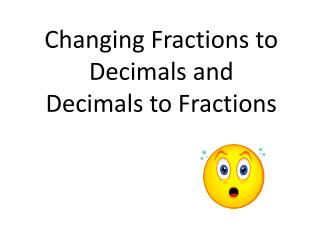DownloadDownload PresentationChanging Fractions to Decimals and Decimals to Fractions

# Changing Fractions to Decimals and Decimals to Fractions

Télécharger la présentation## Changing Fractions to Decimals and Decimals to Fractions

- - - - - - - - - - - - - - - - - - - - - - - - - - - E N D - - - - - - - - - - - - - - - - - - - - - - - - - - -
##### Presentation Transcript

1. Changing Fractions to Decimals and Decimals to Fractions

2. Remember that a fraction is another way to write a division problem… So ¾ means 3 divided by 4. Therefore…

3. To change a decimal to a fraction you need to know your place values… Change 0.45 to a fraction. Read the number… forty-five hundredth

4. Example #1: Change ⅜ to a decimal.

5. Example #2 Change 0.48 to a fraction. That is read forty-eight hundredth. Change 0.48 to a percent. Percents are fractions out of 100. Since this is forty-eight hundredth then 0.48 = 48%

6. When asked to change a repeating decimal to a fraction, you will need to need to… Change to a fraction.

7. Example #3 Change to a fraction.

8. Example #4: Change to a fraction.